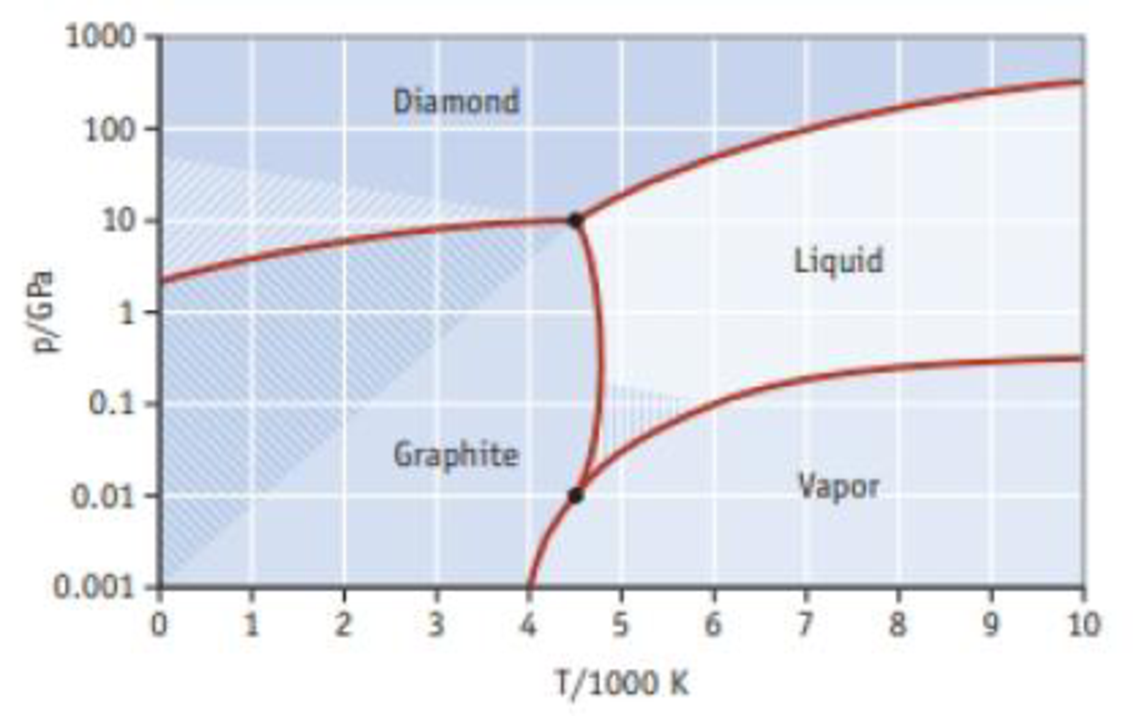Chapter 12, Problem 71SCQ

Chapter
Section
Textbook Problem

Phase diagrams for materials that have allotropes can be more complicated than those shown in the chapter. Use the phase diagram for carbon given here to answer the following questions.(a) How many triple points are present and what phases are in equilibrium for each? (b) Is there a single point where all four phases are in equilibrium? (c) Which is more stable at high pressures, diamond or graphite? (d) Which is the stable phase of carbon at room temperature and 1 atmosphere pressure?

(a)

Interpretation Introduction

Interpretation:

The triple points that are present and phases that are in equilibrium for each have to be identified.

Concept introduction:

Under certain specific conditions, two states can coexist in equilibrium; can be represented in phase diagram.

The triple point of a substance is the temperature and pressure at which the three phases (gas, liquid, and solid) of that substance coexist in thermodynamic equilibrium.

Explanation

Phase diagram is shown here in Figure 1,

(b)

Interpretation Introduction

Interpretation:

A single point where all phases are in equilibrium has to be identified.

Concept introduction:

Under certain specific conditions, two states can coexist in equilibrium; can be represented in phase diagram.

The triple point of a substance is the temperature and pressure at which the three phases (gas, liquid, and solid) of that substance coexist in thermodynamic equilibrium.

(c)

Interpretation Introduction

Interpretation:

Diamond or graphite is more stable at high temperature has to be determined

Concept introduction:

Under certain specific conditions, two states can coexist in equilibrium; can be represented in phase diagram.

The triple point of a substance is the temperature and pressure at which the three phases (gas, liquid, and solid) of that substance coexist in thermodynamic equilibrium.

(d)

Interpretation Introduction

Interpretation:

The stable phase of carbon at room temperature and 1 atmosphere pressure has to be determined.

Concept introduction:

Under certain specific conditions, two states can coexist in equilibrium; can be represented in phase diagram.

The triple point of a substance is the temperature and pressure at which the three phases (gas, liquid, and solid) of that substance coexist in thermodynamic equilibrium.

Still sussing out bartleby?

Check out a sample textbook solution.

See a sample solution

The Solution to Your Study Problems

Bartleby provides explanations to thousands of textbook problems written by our experts, many with advanced degrees!

Get Started